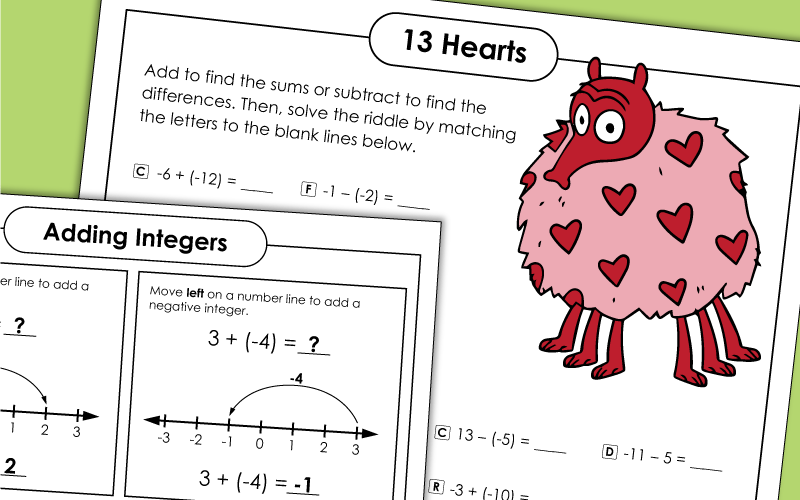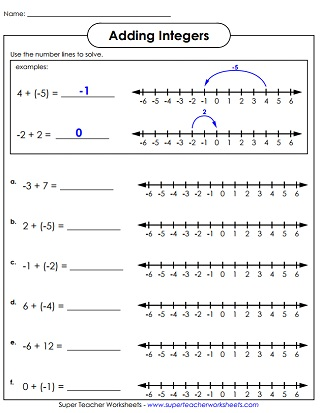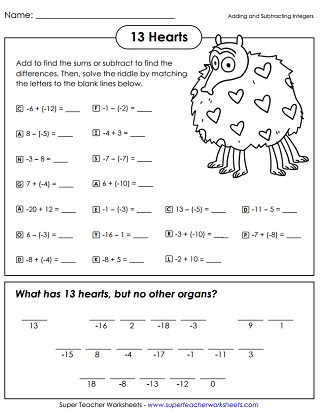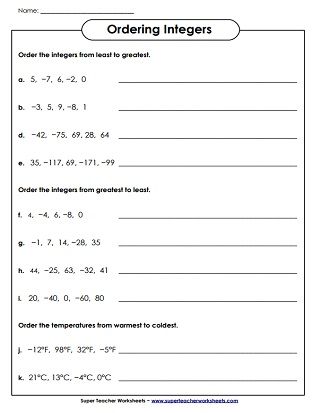# Integers

Integers are positive and negative whole numbers. Teach students about comparing, ordering, adding, subtracting, multiplying, and dividing basic integers.## Basic Integers

Compare positive and negative numbers using the greater than and less than symbols.
Write the positive and negative numbers in order, from least to greatest.
Place the negative and positive integers on the number lines and answer the questions.
For each sentence, fill in the blank line to demonstrate your knowledge of integers.

Learn to add integers using a number line with positive and negative numbers.
Practice adding negative and positive integers on a number line.
At the top of this worksheet, there are many shapes with positive and negative numbers in them. Students find pairs of congruent shapes, and add the numbers inside of them. For example: Find the sum of the numbers in the trapezoids.

## Subtracting Integers

Find out how to subtract using a number line with negative and positive integers.
Practice subtracting negative and positive integers on a number line.

## Adding & Subtracting Integers (Mixed)

What has 13 hearts, but no other organs? To find the answer to the riddle, add and subtract positive and negative numbers. Then match the letters with the answers.

## Multiplying Integers

At the top of this worksheet, students are presented with shapes that have positive and negative integers in them. Students multiply similar shapes together. For example:Find the product of the numbers in the hexagons.
This multiplication table features negative and positive integers ranging from negative four through positive four.

## Mixed Operations

Add, subtract, multiply, and divide integers on this practice worksheet. Includes 13 regular problems and 2 word problems.

## Integer Number Lines

Here's a large number line (-20 to 20) that you can cut out and hang on the wall of your classroom.
Here's a large number line that you can cut out, assemble, and hang on your classroom wall.

Pre-Algebra & Algebra Worksheets

Students will learn to evaluate expressions, solve equations, identify dependent/independent variables, and work with inequalities.

Number Lines

These number line worksheets can be used to teach students about integers, skip counting, addition, subtraction, and number patterns.

## Sample Worksheet ImagesMy Account
Site Information